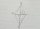# Right triangle + expression of a variable from formula - problems

1. Ratio of sidesCalculate the area of a circle that has the same circumference as the circumference of the rectangle inscribed with a circle with a radius of r 9 cm so that its sides are in ratio 2 to 7.
2. Lateral surface areaThe ratio of the area of the base of the rotary cone to its lateral surface area is 3: 5. Calculate the surface and volume of the cone, if its height v = 4 cm.
3. A kiteABCD is a kite. Angle OBC = 20° and angle OCD = 35°. O is the intersection of diagonals. Find angle ABC, angle ADC and angle BAD.
4. Rectangular trapezoidIn a rectangular trapezoid ABCD with right angles at vertices A and D with sides a = 12cm, b = 13cm, c = 7cm. Find the angles beta and gamma and height v.
5. RT sidesFind the sides of a rectangular triangle if legs a + b = 17cm and the radius of the written circle ρ = 2cm.
6. Right ΔA right triangle has the length of one leg 28 cm and length of the hypotenuse 53 cm. Calculate the height of the triangle.
7. Cone A2VSurface of cone in the plane is a circular arc with central angle of 126° and area 415 cm2. Calculate the volume of a cone.
8. Square diagonalCalculate length of the square diagonal if the perimeter is 476 cm.
9. IS trapezoidCalculate the length of diagonal u and height v of isosceles trapezoid ABCD, whose bases have lengths a = |AB| = 37 cm, c = |CD| = 29 cm and legs b = d = |BC| = |AD| = 28 cm.
10. Floating barrelBarrel (cylinder shape) floats on water, top of barrel is 8 dm above water and the width of surfaced barrel part is 23 dm. Barrel length is 24 dm. Calculate the volume of the barrel.
11. R TrapeziumRectangular trapezium has bases 12 and 5 and area 84 cm2. What is its perimeter?
12. ISO triangleCalculate the area of an isosceles triangle KLM if the length of its sides are in the ratio k:l:m = 4:4:3 and has perimeter 377 mm.
13. Rhombus 2Calculate the area of rhombus which has a height v=48 mm and shorter diagonal u = 60 mm long.
14. Right isoscelesCalculate area of the isosceles right triangle which perimeter is 41 cm.
15. Square diagonalCalculate the length of diagonal of the square with side a = 23 cm.
16. Right triangleCalculate the missing side b and interior angles, perimeter and area of ​​a right triangle if a=10 cm and hypotenuse c = 16 cm.
17. Perimeter and legsDetermine the perimeter of a right triangle if the length of one leg is 75% length of the second leg and its content area is 24 cm2.
18. Cone and the ratioRotational cone has a height 23 cm and the ratio of the base surface to lateral surface is 7: 9. Calculate a surface of the base and the lateral surface.
19. Cathethus and the inscribed circleIn a right triangle is given one cathethus long 14 cm and the radius of the inscribed circle of 5 cm. Calculate the area of this right triangle.
20. Nice prismCalculate the surface of the cuboid if the sum of its edges is a + b + c = 19 cm and the body diagonal size u = 13 cm.

Do you have an interesting mathematical problem that you can't solve it? Enter it, and we can try to solve it.

To this e-mail address, we will reply solution; solved examples are also published here. Please enter e-mail correctly and check whether you don't have a full mailbox.